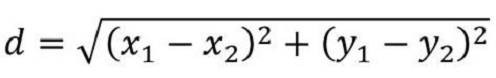# Finding Euclidean distance using Scikit-Learn in Python

In this article, we will learn to find the Euclidean distance using the Scikit-Learn library in Python.

### Methods Used

• Calculating Euclidean Distance using Scikit-Learn

• Calculating Euclidean Distance Between Two Arrays

For machine learning in Python, Scikit-Learn is the most effective and useful library. Regression, classification, clustering, and other useful machine learning methods are among the many tools it contains. Euclidean distance is one of the metrics that clustering algorithms employ to determine how well the clusters have been optimized i.e, the degree of optimization of the clusters.

The well-known Distance Formula in two dimensions has been used by all of us to determine the distance between two points in geometry −

Euclidean Distance Formula −where, (x1, x2) and (x2, y2)- are the points on the cartesian plane.

## Method 1: Calculating Euclidean Distance using Scikit-Learn

### Algorithm (Steps)

Following are the Algorithm/steps to be followed to perform the desired task. −

• Use the import keyword to import the euclidean_distances() function from the sklearn module.

• Use the import keyword to import the NumPy module with an alias name np.

• Use the numpy.array() function to create a NumPy array and give random array elements to it.

• Use the euclidean_distances() function to calculate the euclidean distance between the given NumPy array elements(coordinates) and the origin(0,0,0) by passing the input array, and the origin list as arguments to it.

• Print the resultant euclidean distance.

### Example

The following program returns the euclidean distance between array elements and origin using the euclidean_distances() function of the sklearn module −

# importing euclidean_distances function from scikit-learn module
from sklearn.metrics.pairwise import euclidean_distances
# importing NumPy module with an alias name
import numpy as np
# input NumPy array
inputArray = np.array([[3.5, 1.5, 5],
[1, 4, 2],
[6, 3, 10]])
#calculating the euclidean distance between the given NumPy Array and Origin(0,0)
resultDistance = euclidean_distances(inputArray, [[0, 0, 0]])
# printing the resultant euclidean distance
print("Resultant euclidean distance:\n", resultDistance)


### Output

On executing, the above program will generate the following output −

Resultant euclidean distance:
[[ 6.28490254]
[ 4.58257569]
[12.04159458]]


## Method 2: Calculating Euclidean Distance Between Two Arrays

The Euclidean Distance between two array elements can be calculated in the same way. Therefore, if the lists have m and n elements, respectively, the output array will have m * n elements.

### Algorithm (Steps)

Following are the Algorithm/steps to be followed to perform the desired task. −

• Use the import keyword to import the euclidean_distances function from sklearn module.

• Use the import keyword to import the numpy module with an alias name.

• Use the numpy.array() function to create a first NumPy array and create a variable to store it.

• Use the numpy.array() function to create a second NumPy array and create another variable to store it.

• Use the euclidean_distances() function to calculate the euclidean distance between the given two input array elements by passing the input array 1, and input array 2 as arguments to it.

• Print the resultant euclidean distance.

### Example

The following program returns the euclidean distance between two corresponding input array elements using the euclidean_distances() function of the sklearn module −

# importing euclidean_distances function from
# scikit-learn module
from sklearn.metrics.pairwise import euclidean_distances
# importing numpy library with an alias name
import numpy as np
# input numpy array 1
inputArray_1 = np.array([[3.5, 1.5, 5],
[1, 4, 2],
[6, 3, 10]])
# input numpy array 2
inputArray_2 = np.array([[5, 4, 2],
[4, 3, 1],
[8.5, 2, 6]])
# calculating the euclidean distance between inputArray_1 and inputArray_2
resultDistance = euclidean_distances(inputArray_1, inputArray_2)
# printing the resultant euclidean distance
print("Resultant euclidean distance:\n", resultDistance)


### Output

On executing, the above program will generate the following output −

Resultant euclidean distance:
[[4.18330013 4.30116263 5.12347538]
[4.         3.31662479 8.7321246 ]
[8.1240384  9.21954446 4.82182538]]


The output, as we can see, is a 2D array. Each item in this array contains the distance between a point in the first array, labeled as "inputArray_1" and the other set of points in the second array, designated as "inputArray_2".

### What role does Euclidean distance have in clustering algorithms?

A sort of unsupervised machine learning method called clustering algorithms divides a dataset into groups (referred to as clusters) depending on how similar the data is to one another. Euclidean distance is frequently used to compare the similarity of data points, with closer points being seen as more similar. The distance between points in a clustering method is used to choose which points belong in the same cluster. This may be achieved by computing the Euclidean distance between each pair of points and selecting the points that should be grouped together based on a threshold value. As an alternative, clustering algorithms can compute the centroid of a cluster, which is the average location of all the points in the cluster, using the Euclidean distance between points. This may be used to update the cluster's point locations and increase the clustering algorithm's accuracy.

## Conclusion

Using the sklearn module's euclidean distances() function, we learned how to calculate euclidean distances in this article. We took two examples to demonstrate in a clear way i.e Finding the euclidean distance from a point to the origin and Finding the euclidean distance between two points.

Updated on: 01-Feb-2023

2K+ Views